# computer architecture assignment Question #2 Design a 4-bit subtractor circuit.

computer architecture assignment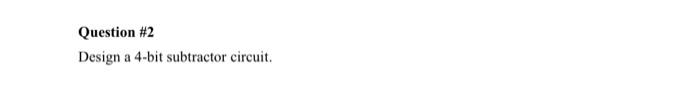Question #2 Design a 4-bit subtractor circuit.

This is the binary adder /subtractor circuit.

Page-1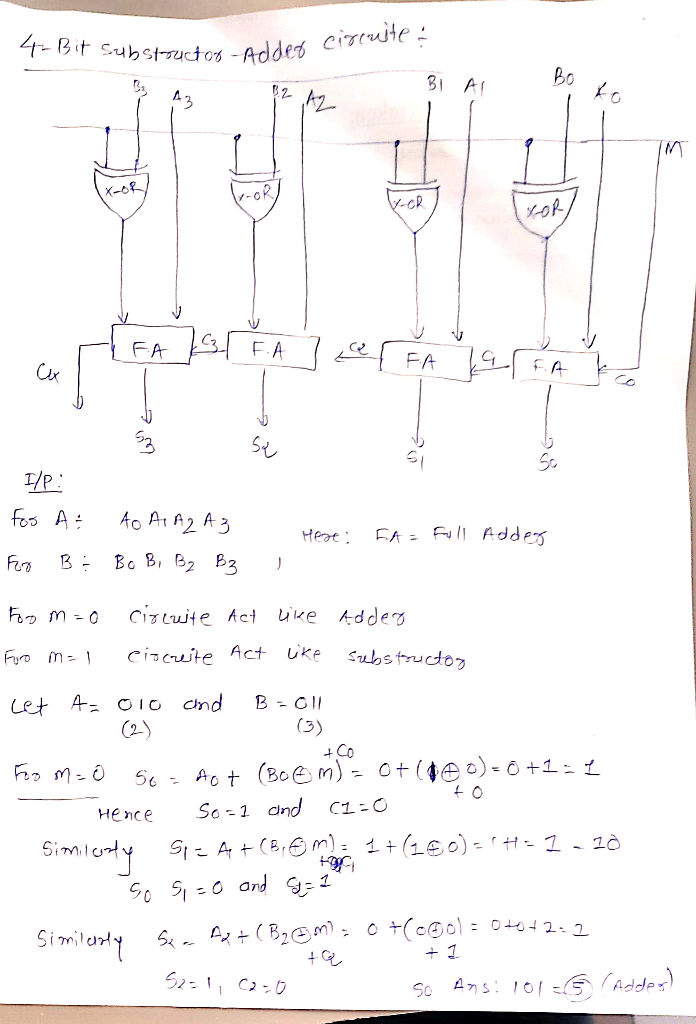Page-2##### Add Answer to: computer architecture assignment Question #2 Design a 4-bit subtractor circuit.
Similar Homework Help Questions
• ### 4. Design a 4-bit Adder / Subtractor. Follow the steps given below. (a) Write the VHDL...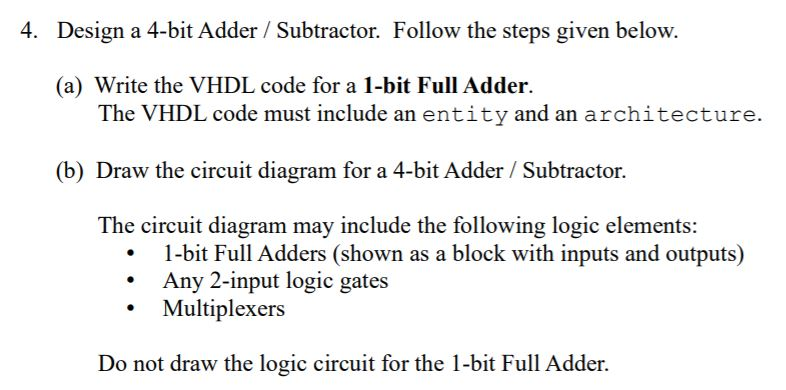4. Design a 4-bit Adder / Subtractor. Follow the steps given below. (a) Write the VHDL code for a 1-bit Full Adder. The VHDL code must include an entity and an architecture. (b) Draw the circuit diagram for a 4-bit Adder / Subtractor. The circuit diagram may include the following logic elements: 1-bit Full Adders (shown as a block with inputs and outputs) Any 2-input logic gates Multiplexers Do not draw the logic circuit for the 1-bit Full Adder.

• ### Computer Architecture Design Question

From Computer Systems Organization &Architecture - JohnCarpinelli (author)Similar to Chapt. 8 - question #29. pg. 375.Show the contents of a lookup ROM that implements a 4-bit x4-bit multiplier.

• ### (1) How do you design a 4-bit subtractor (i.e. C = A - B) using 1-bit...

(1) How do you design a 4-bit subtractor (i.e. C = A - B) using 1-bit full adders, and with circuits for overflow detection. (Note: output 1 if there is overflow; otherwise, output 0.)

• ### 3. Design a one bit subtractor. The circuit subtracts the number Y from X and generates...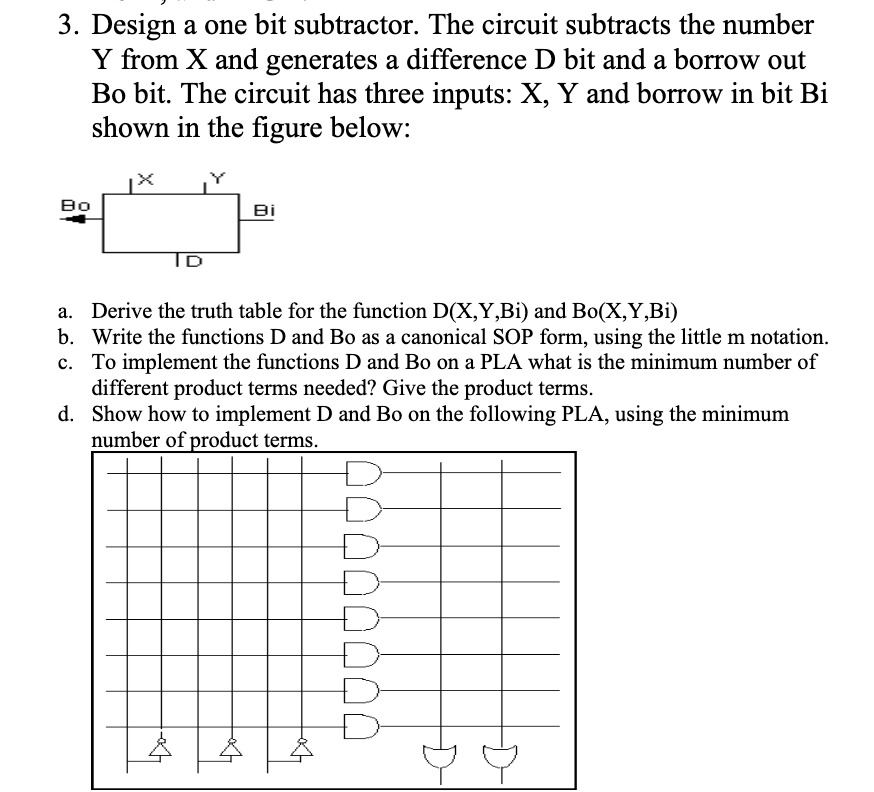3. Design a one bit subtractor. The circuit subtracts the number Y from X and generates a difference D bit and a borrow out Bo bit. The circuit has three inputs: X, Y and borrow in bit Bi shown in the figure below: во ID a. Derive the truth table for the function D(X,Y,Bi) and Bo(X,Y,Bi) b. Write the functions D and Bo as a canonical SOP form, using the little m notation. c. To implement the functions D and...

• ### 2-bit subtractor

Develope with clear explanations and reasoning a 2-bit subtractor, namely for the 15th and 16th bit of a 16-bit subractor. Show the truth table, K-map etc.. Keep inmind, there is no borrow in, so the truth table has ony 4 variables

• ### Construct the 8-bit ripple-carry adder/subtractor for signed integers. Negative numbers are in th...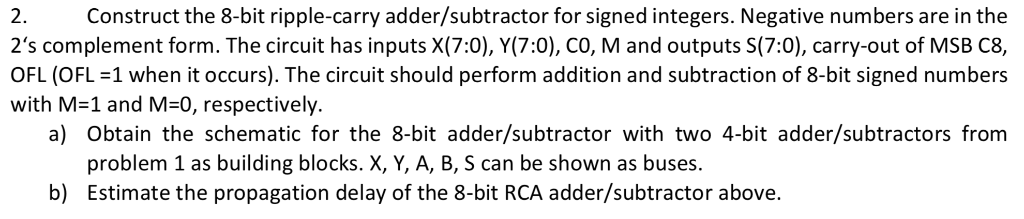Construct the 8-bit ripple-carry adder/subtractor for signed integers. Negative numbers are in the 2's complement form. The circuit has inputs X(7:0), Y(7:0), CO, M and outputs S(7:0), carry-out of MSB C8, OFL (OFL 1 when it occurs). The circuit should perform addition and subtraction of 8-bit signed numbers 2. with M-1 and M-0, respectively. a) Obtain the schematic for the 8-bit adder/subtractor with two 4-bit adder/subtractors from problem 1 as building blocks. X, Y, A, B, S can be shown...

• ### Designing a 2's complement overflow circuit

>>>this problem is confusing, do not know how to start.A Subtractor takes two 4-bit inputs A3:0 and B3:0 , performs A - B, outputs difference D3:0and borrow E4:1. Design a 2's complement overflow detection circuit for this Subtractor by following the steps.NOTE that you are NOT askedto design a 4-bit Subtractor.Step 1, identify the inputs needed by the 2's complement overflow detection circuit and draw itstruth table.Step 2, find its minterm expansion

• ### I am trying to find out a design containing NOR and OR or AND gate for the interface block design...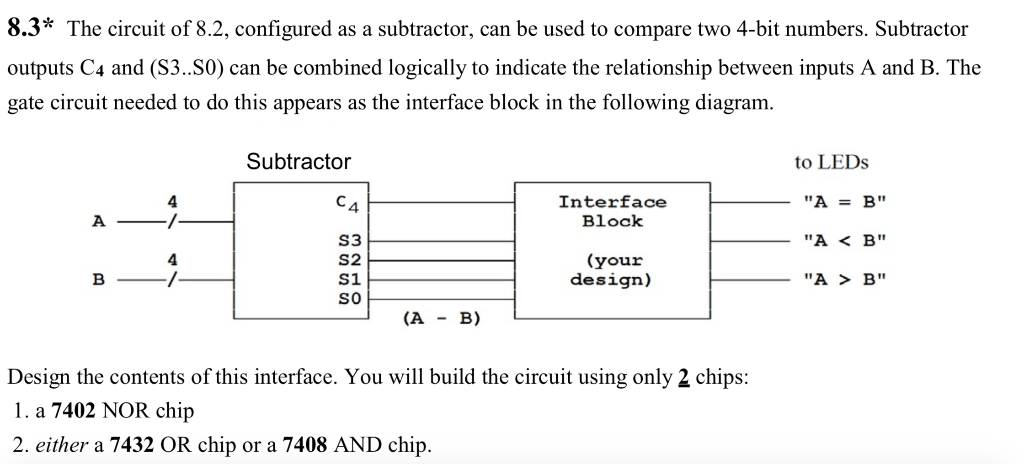I am trying to find out a design containing NOR and OR or AND gate for the interface block design. I have completed a design for when A = B, but I am having difficulty designing A < B and B > A on the subtractor where the boxes have question marks. Please help! 8.3* The circuit of 8.2, configured as a subtractor, can be used to compare two 4-bit numbers. Subtractor outputs C4 and (S3..S0) can be combined logically...

c) Draw the schematic of an Adder/Subtractor unit using 4-bit binary adder andsome external gates, which gives out A+B if C=0 and A-B if C=1 as in the ALU designof Lab # 4. Also provide an indicator for checking the overflow? Use the above designedcircuit as block box and give a scheme for finding the absolute value of a 4-bit number?Draw all the input/output signals clearly in your design schematic.

• ### Design a combinational circuit that compares two 4-bit unsigned numbers A and B

Design a combinational circuit that compares two 4-bit unsigned numbers A and B to see whether B is greater than 2A. The circuit has one output X, so that:X = 1 if 2A < B, andX = 0 if 2A >= B.Please de descriptive and detailed in drawing.The book we are using in class is this: http://www.cramster.com/logic-and-computer-design-fundamentals-4th-solutions-3631 and we are on chapter 4.

Free Homework App